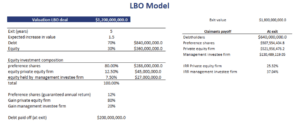# LBO Model

The LBO model, or LBO valuation model is a method for factoring in the company’s capital structure and other parameters when determining the return on equity a private equity firm can expect from an LBO transaction. But what is an LBO model? Unlike many other approaches used in corporate finance, the model is not used to determine the value of a company. Rather, the LBO model is used to determine the maximum price that a private equity company should be will to pay. Thus, the resulting value is used during the negotiations.

On this page, we discuss the basic elements of an Leveraged Buyout Model and provide an LBO model Excel template. In the Excel spreadsheet, we develop a small Leveraged Buyout Model model example.

## LBO model template

A basic LBO model template consists of three inputs or steps:

1. the target company’s (TC) forecasted CFs
2. expected returns required by the financiers
3. total amount of financing needed

From this information, we can determine the way the funding for the deal can be structured. Once we have that information, we calculate the likely return to the different participants in the LBO deal.

## LBO model example

Let’s get a sense of how to use the model using a simple LBO model walkthrough. Admittedly, the discussion above may too vague for many to be able to apply the approach. Fortunately, we can easily shed more light on the topic by considering a simple example.

The following table illustrates how the model can be used to analyze an LBO transaction. In this excercise we calculate the payoff multiple the different participants to the transaction can expect to earn, assuming a certain exit value. This example can easily be extended to account for other types of financing.This simple LBO model Excel template can be downloaded at the bottom of the page. It shows that we can easily estimate the likely return on investment of the different parties involved in the deal. The return on investment will, of course, depend crucially on the exit value. The latter has to be estimated and is subject to considerable estimation error.

What is important to understand about the Leveraged Buyout Model model is that we determine the maximum value we are will pay today. That is, the maximum price considering the return we require or expect on the investment as a private equity investor.

This is a very different perspective than the one used in more traditional valuation models such as a dividend discount model. When using such models, the results is a price per share that is assumed to be the appropriate price an investor should pay for the firm.

## Summary

We discussed the Leveraged Buyout Model, a useful way to look at an LBO transaction. Unlike many other models in corporate finance, the model should not be used for valuation. Rather, the model should be used to determine the maximum price the private equity is willing to pay.

### Leveraged buyout model Excel Template

Want to have an implementation in Excel? Download the Excel file: LBO Model